##### Actions

A linear topology of a ringin which the fundamental system of neighbourhoods of zero consists of the powers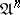of some two-sided ideal. The topology is then said to be-adic, and the idealis said to be the defining ideal of the topology. The closure of any set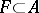in the-adic topology is equal to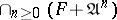; in particular, the topology is separable if, and only if,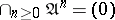. The separable completionof the ringin an-adic topology is isomorphic to the projective limit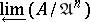.
The-adic topology of an-moduleis defined in a similar manner: its fundamental system of neighbourhoods of zero is given by the submodules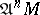; in the-adic topologybecomes a topological-module.
Letbe a commutative ring with identity with an-adic topology and let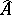be its completion; ifis an ideal of finite type, the topology inis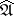-adic, and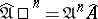. Ifis a maximal ideal, thenis a local ring with maximal ideal. A local ring topology is an adic topology defined by its maximal ideal (an-adic topology).
A fundamental tool in the study of adic topologies of rings is the Artin–Rees lemma: Letbe a commutative Noetherian ring, letbe an ideal in, let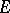be an-module of finite type, and let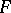be a submodule of. Then there exists asuch that, for any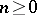, the following equality is valid: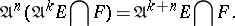The topological interpretation of the Artin–Rees lemma shows that the-adic topology ofis induced by the-adic topology of. It follows that the completionof a ringin the-adic topology is a flat-module (cf. Flat module), that the completion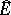of the-moduleof finite type is identical with, and that Krull's theorem holds: The-adic topology of a Noetherian ring is separable if and only if the set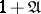contains no zero divisors. In particular, the topology is separable ifis contained in the (Jacobson) radical of the ring.